# than rigid-face irons

Dave Tutelman  --  March 4, 2009

On Nov 30, 2008, I had a debate on the Spinetalker's forum with Tom Wishon and Richard Kempton. The subject was high-COR irons. That is, irons whose faces flex enough to give a spring effect, which increases the Coefficient Of Restitution (COR) and raises the ball speed. This is not an idle nor hypothetical discussion; Tom Wishon Golf Technology (TWGT) offers such an iron, the 770cfe, and is introducing another model this month.

Here were the arguments:
• My point: while drivers and 3-woods are about maximum distance, irons need to be about repeatable, dependable distance. It's OK if I can't hit my 7-iron as far as you can hit yours, but it's a disaster if I don't know how far my 7-iron shot is going to go.
• Tom's point: there are lots of golfers who would love to hit their irons longer. Distance sells. Older golfers want to get back the distance they lost. Etc, etc, etc.
• My point: There is going to be some falloff of COR as the strike moves away from the center of the clubface. With good design there may not be much, but there will be some. So that adds to the loss already there for an off-center hit. Yes there is loss even for a rigid clubface, due to clubhead rotation because the moment of inertia is not infinite. Adding to this loss with COR falloff further increases the uncertainty of how far the ball will travel.
• Richard's point: based on his experience, his customers' experience, and Tom's assurances, there is no more loss of distance with the 770cfe than with rigid face irons. In fact, they were even more forgiving of an off-center strike.
 I was skeptical of that last claim. This was my reasoning at the time...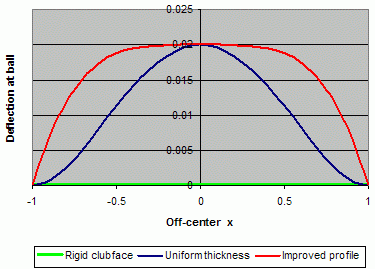The COR of a rigid face club and a modern ball is about 0.77. COR increases with the face flex. The COR boost due to spring effect is roughly proportional to the amount the face deflects where the ball strikes it. Let us look at the face deflection for a few possible clubface designs. The green curve is the rigid clubface. It is just a flat line at zero -- obviously, because "rigid" means the clubface does not deflect. So there is no COR boost for this face; the COR is stuck on 0.77. The dark blue curve is a uniformly thin face. It flexes a lot in the center, but the COR boost falls off fairly quickly as the hit misses the center. This would not send the ball a reliable distance, because the COR would drop off towards 0.77 quickly if the strike was not on-center. The red curve depicts what I felt could reasonably be done by selectively thickening the face and changing it flex characteristics.This retains most of the high COR for some degree of off-center hit, but falls off if you get a bit farther from the center. Remember that the goal is to make the club as forgiving as possible, in the sense that the distance does not change much in the event of a mishit. Of the three curves shown, the rigid face is the best at meeting this criterion. No, it does not give maximum distance, but it does give the least loss of distance for an off-center hit. The red curve is also pretty good, as long as you don't miss the center by too much, but still loses a lot of distance for a significantly off-center hit. And it was my belief that the red curve was about the best you could do in this regard. Let's remember that there is another reason we lose distance when we miss the center of the clubface, even with a perfectly rigid clubface -- clubhead rotation. A high clubhead moment of inertia can minimize this loss, but even a high-MOI clubhead will show significant loss if the strike is significantly off-center. So whatever "rolloff" of ball speed we get from off-center reduced deflection is on top of the loss from clubhead rotation.

I continued the discussion with Richard off-list. We came to the conclusion that, for the 770cfe to be more forgiving (not just longer), at least one of the following had to be true:
1. The MOI of the clubhead is greater than the normal iron. This was certainly possible, because the thin portions of the face provide a bit of "discretionary mass" to be moved to the periphery. But Tom insisted that the bulk of the difference was in COR. So we are left with...
2. The face is designed such that the COR increases (not decreases) as the impact point moves away from the center. That is, the deflection of the face at the point of impact must be more away from the center than at the center. This is not just a constant COR (like a rigid clubface), but a COR that curves upwards away from the center -- totally unlike the red or dark blue curves above.
I was very skeptical that this could be accomplished. I thought that, to resolve the question, I would have to gear up to analyze the deflection of a non-uniform beam with a non-centered load. While not terribly difficult conceptually, it is a lot of work if you don't have structural design software or finite-element analysis (FEA) software. I don't have software packages like that.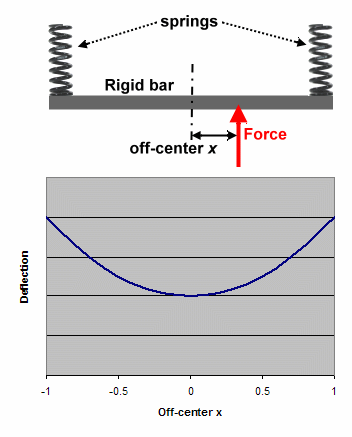But then I came up with a remarkably simple model that did exactly what Richard and I needed; it increased deflection as the point of impact moved away from the center. Consider the system shown in the diagram. A rigid bar is supported by two identical springs, one at each end. A force (representing the impact force of the ball) is applied somewhere along the bar, x distance from the center.

Let's start with an intuitive explanation of the behavior of this system; then we'll proceed with an actual analysis.
• If the force is dead center (x=0), then each spring gets to resist half the force.
• If the force is at either end (x= ±1), then the entire force is resisted by just the spring at that end. So the bar -- where the force is applied -- experiences twice the deflection.
• Between the center and the ends, we would expect some sort of smooth variation. This is not a for-sure given, but there is no reason to expect otherwise. And the analysis will bear it out.
If you're interested, the analysis is at the end of this article. The algebra is more work than the physics, and there isn't much to the algebra either. When you're all done, the function for the deflection is very simple:
 deflection  = F 2K (x2 + 1)
where F is the force and K is the spring constant of each spring. You can see the deflection function on the graph.

So, at least for the length of the rigid bar, deflection (and thus COR) is an increasing function of distance from the center. Not only that -- it is a square-law function. Why is that important? Because the loss of ball speed from clubhead rotation on an off-center hit is also square-law. So it should not only be possible, but easy, for COR to compensate nearly exactly for MOI losses. This was a big surprise to me.

But there is still a bit of a problem. This model does not look anything like a clubhead. It certainly does not look anything like a TWGT 770cfe iron head. And it looks like just the sort of kludge the USGA would be ready to ban instantly. How do we turn this physics existence proof into a golf club?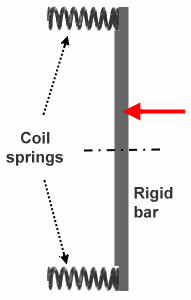The next few diagrams show the evolution of the concept from physics textbook to the Wishon catalog.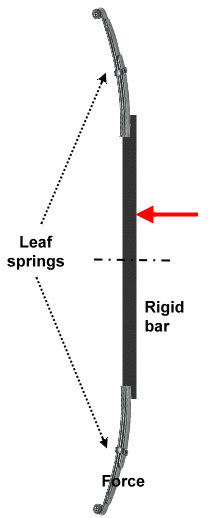We start with the original version of the system. Then we remember that coil springs are not the only kind of spring available. The second figure has replaced the coil springs with leaf springs, like those that allow the wheels of a car to soak up bumps in the road. But what are leaf springs? They are just thinner, more flexible pieces of steel. If they were the same steel and the same thickness as the rigid bar in the diagram, then they would be more of a rigid bar, and not springs at all. So we could spring the bar by simply narrowing it to a thin strip of the same material at the ends. Of course, the bar isn't perfectly rigid; there will be some small amount of flex in it. But compared to the thin section at the end the thick section looks rigid -- and the thin section looks like a spring. It is still the same as the original model with the coil springs and the rigid bar, to the tolerances that matter in a golf club.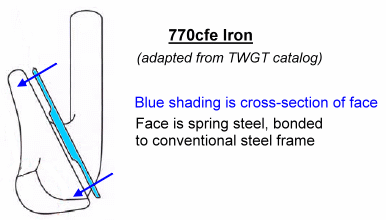And that is essentially what Tom Wishon has done. The third figure is a picture adapted from the 2009 TWGT catalog. The face is a separately fabricated insert, shown in cross-section and color-coded in blue. It has a thick (read that as rather rigid) portion in the middle, and thinner flanges that connect it to the conventional rigid steel frame. But the thin flanges are much wider than they need to be just to connect to the frame. There is enough thin area there to be a spring edge for the rigid center. So the thick center acts as the rigid bar, and the thin edge acts as the leaf spring. As long as you hit somewhere on the thick part, you will see the spring behavior where increasing COR makes up for the loss from clubhead rotation. If the hit is so far off-center that it is on the thin portion, you will still get some "save" out of it -- though the COR must necessarily fall as the strike approaches the conventional, rigid frame supporting the periphery of the face.

So let me compliment Tom on a really innovative design. Richard says it does what it intends, and now I have no reason to doubt it.

## Analysis

For those who want to see how we got the graphs, here is the physics and math behind the curves.

### Deflection of uniform beam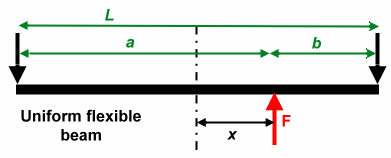Let us find the deflection of a uniform flexible beam, loaded with a force F at a distance x from the center. We want the deflection at x, which is where the ball impact would be if it were a clubface. We use the well-known formula
 deflection  = Fab6EIL (L2 - a2 - b2) = Fab6EIL ([a+b]2 - a2 - b2) = Fab6EIL (a2 + 2ab + b2 - a2 - b2) = Fab6EIL (2ab) = Fa2b23EIL
Let us normalize everything so there is a unit distance between the center and either end. So L=2. That means that a=1+x and b=1-x. Substituting back into the equation for deflection gives
 deflection = F (1+x)2 (1-x)26EI = F (1-x2)26EI

### Deflection of rigid beam with springs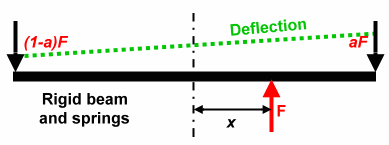Let us find the deflection at x of a rigid bar suspended at the ends by two springs of spring constant K. In this case, the deflection is not the bending of the beam, but rather the motion of the beam restrained by the springs.

The forces at the ends must equal F for equilibrium. Let us denote them as aF and (1-a)F. (We will worry later about the value of a. Let's just use it for now in the deflection curve.)

Since the beam is a rigid bar, the deflection is a straight line (the green dotted line in the diagram). It goes from the spring deflection at the left to the spring deflection at the right. We will normalize the length again, so the line goes from -1 to +1. If we call the distance along the bar t (because x is already taken to mean the position of the force), then the deflection at t=-1 is (1-a)F/K and at t=+1 is aF/K.

We can get the equation for the deflection curve by the slope-intercept method I remember from tenth grade. The intercept is the average of the two end deflections, or F/2K. The slope needs a little more work.
 slope  = aF - (1-a)F2K = F (2a - 1)2K
So the slope-intercept equation (using t for the x-axis index) is
deflection  =
 F2K (2a - 1)t  + F2K
=
 F2K {(2a - 1)t  +  1}
Next, we want to find a in terms of x, the off-centeredness of the hit. If we balance moments around the left end of the bar, the torque equation is
(1+x)F  =  2aF
 a  = 1+x2

Plugging this back into the equation for the deflection curve, we get
 deflection  = F2K {(2 1+x2 -1) t  +  1}  = F2K (xt + 1)
We are interested in the deflection at the point where the force is, so we evaluate at t=x and get
 deflection  = F2K (x2 + 1)